Welcome to Wall Street Prep! Use code at checkout for 15% off.# The Paper LBO

Guide to Understanding the Paper LBO (Step-by-Step)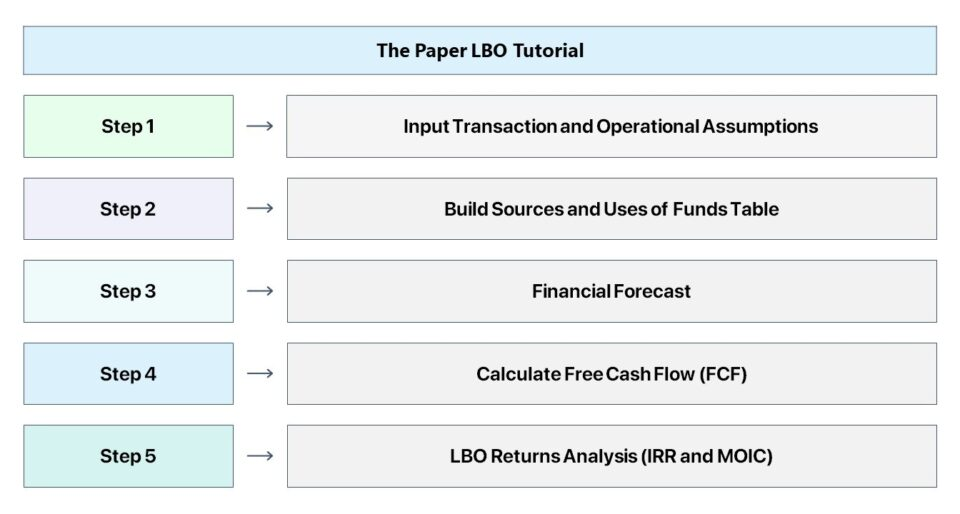## The Paper LBO: Practice Tutorial Guide

Starting off, the interviewee typically receives a “prompt” – a short description containing a situational overview and certain financial data for a hypothetical company contemplating an LBO.

The interviewee will be given a pen and paper and 5–10 minutes to arrive at the implied IRR and other key metrics based exclusively on the information provided in the prompt.

For practically all private equity interviews, do not expect the interviewer to hand you a calculator, because only a pen and paper will be provided.

In fact, the entire paper LBO test could even just be a verbal discussion with the interviewer.

Therefore, make sure to practice completing mental math in your head until you are comfortable performing shorthand calculations under timed pressure.

The paper LBO test is used by most private equity firms – or in some cases, headhunters – to quickly vet a potential candidate and take place at fairly early stages of the PE interview process, i.e. the first round.

As candidates progress to subsequent rounds, private equity firms often ask interviewees to complete a far more detailed LBO modeling test, or perhaps even a take-home case study.

## How to Complete the Paper LBO?

Before we begin, the steps to complete a paper LBO test are outlined below.

• Step 1 → Input Transaction and Operating Assumptions
• Step 2 → Build Sources and Uses of Funds Table
• Step 3 → Project Financials
• Step 4 → Calculate Free Cash Flow (FCF)
• Step 5 → LBO Returns Analysis

## Illustrative Paper LBO Prompt

To get started, the following is an example prompt for our practice paper LBO test.

###### Paper LBO Prompt Example

JoeCo, a coffee company, has generated \$100mm in last twelve months (LTM) revenue and this figure is expected to increase by a growth rate of 10% annually into the foreseeable future.

JoeCo’s LTM EBITDA was \$50mm and its EBITDA margin should remain unchanged in the years ahead.

Based upon management guidance, JoeCo’s depreciation and amortization (D&A) and its capital expenditures (Capex) is expected to be 5.0% as a percentage of revenue, with no change in net working capital (NWC) and the effective tax rate fixed at 25%.

If a PE firm acquired JoeCo for 10.0x EBITDA and exited at the same multiple five years later, what is the implied internal rate of return (IRR) and multiple on invested capital (MOIC)?

For the financing of the LBO, assume the initial leverage ratio used to fund the purchase was 6.0x EBITDA and that the debt carries an interest rate of 8.0% with no required principal amortization until maturity, at which debt is fully paid down upon exit.

## Paper LBO Example

Fill out the following form to access the paper LBO template, which we recommend using to confirm your calculations are correct.

However, it is rather unlikely that you’ll receive a laptop with Excel in the interview setting, nor a calculator.

Therefore, we print out the 1st sheet to solve this problem set and complete the exercise using pen and paper to practice under the actual testing conditions.#### Excel TemplateSubmitting...

## 1. Input Transaction and Operating Assumptions

The first step is to lay out the operational assumptions that were provided in the prompt and to calculate the total amount paid to purchase the target company.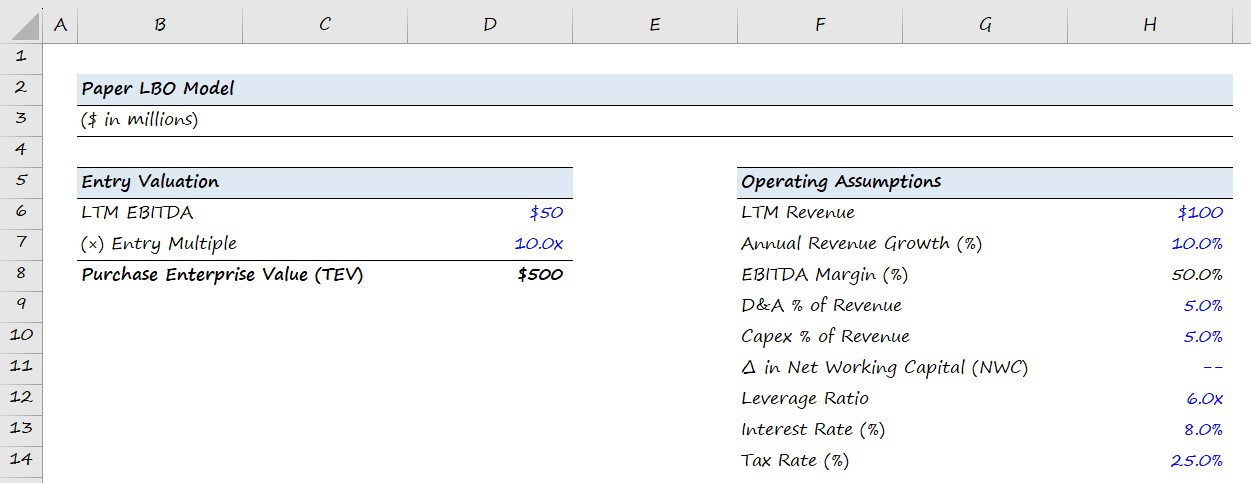Purchase Enterprise Value (TEV) = LTM EBITDA × Entry Multiple
EBITDA Margin (%) = LTM EBITDA ÷ LTM Revenue

## 2. Build Sources and Uses of Funds Table

In the next step, we will build out the Sources and Uses table, which will be a direct function of the transaction structure assumptions. In this particular example, the purchase multiple used was 10.0x EBITDA and the deal was funded using 6.0x leverage.

More specifically, the objective of this section is to figure out the exact cost of purchasing the company, and the amount of debt and equity financing that would be required to complete the acquisition.

The amount of debt used will be calculated as a multiple of LTM EBITDA, while the amount of equity contributed by the private equity investor will be the remaining amount required to “plug” the gap and make both sides of the table balance.

Ultimately, the main goal of an LBO model is to determine how much the firm’s equity investment has grown, and to do so – we need to first calculate the size of the initial equity check by the financial sponsor.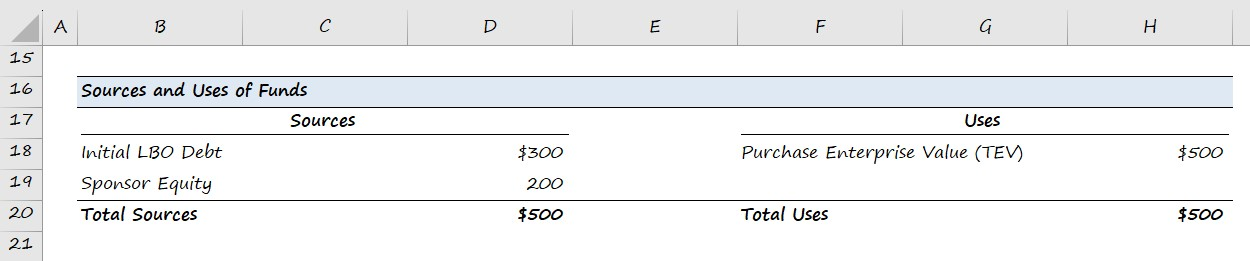Debt Financing = Leverage Multiple × LTM EBITDA
Sponsor Equity = Total Uses Debt Financing

In a real LBO model, the Uses of Funds Section will likely include transaction and financing fees, among other uses. In addition, other more complex concepts like management rollover will be reflected in both the sources and uses of funds.

However, these nuances are unlikely to show up here, so unless you were explicitly provided with additional data in the prompt, focus exclusively on the data provided.

## 3. Financial Forecast

Since we have completed filling out the Sources and Uses of Funds section of our LBO model, we will shift to forecasting the financials of JoeCo from revenue to net income (the “bottom line”).

The operational assumptions that will drive the projections were provided in the first step.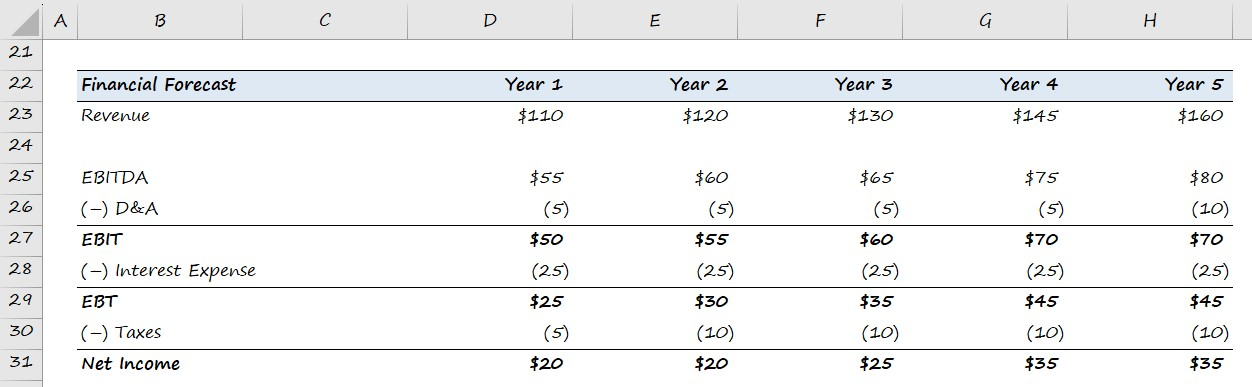Note: In the context of a private equity interview, it is reasonable to round your calculations to the nearest whole number for convenience, i.e. round the figures to the nearest 5 or 10 to simplify the math.

Revenue = Prior Period Revenue × (1 + Annual Revenue Growth Rate)
EBITDA = EBITDA Margin % × Current Period Revenue
D&A = D&A % of Revenue × Current Period Revenue
Interest Expense = Debt Financing Amount × Interest Rate %

## 4. Calculate Free Cash Flow (FCF)

With our financial forecast complete, we can now calculate JoeCo’s free cash flows (FCFs) throughout the five-year holding period.

The FCF generation of an LBO target determines the amount of debt that can be paid down during the holding period. However, our prompt mentioned assume no principal paydown.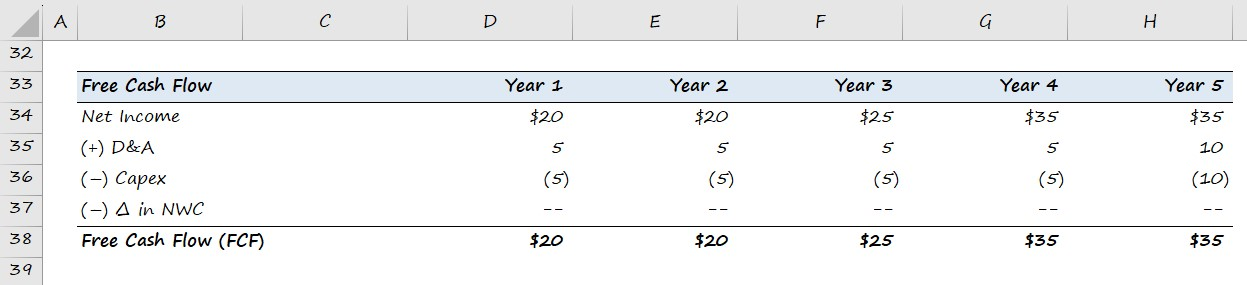Free Cash Flow (FCF) = Net Income + D&ACapexChange in NWC

## 5. LBO Exit Valuation and Return Metrics (IRR and MOIC)

In the last step, we will assess the returns of the investment using the multiple on invested capital (MOIC), or “cash-on-cash return“, and the internal rate of return (IRR).

Earlier, the prompt stated that the PE firm exited the investment at the same multiple as the entry multiple (i.e. no “multiple expansion”).

Since you will likely not have access to a calculator, calculating the IRR requires some “back-of-the-envelope” math.

The standard investment holding period assumption is 5 years, so we recommend memorizing the IRRs based on the most common MOIC returns.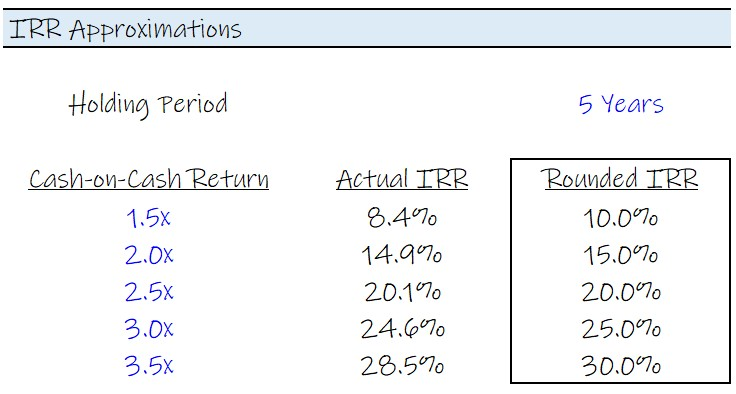###### The Rule of 72 in Paper LBOs

Forgot your IRR approximations? No problem – in most cases, the return should be easy to approximate under the Rule of 72, which estimates the time that it takes to double an investment.

The approximate number of years necessary for the investment value to double in size can be determined by dividing 72 by the rate of return.

For example, over a 5-year horizon, the approximate IRR required to double the investment is ~15%.

• Number of Years to Double = 72 ÷ 5 = ~15%

There’s also the lesser-known Rule of 115, which estimates the time it takes to triple an investment. Here, the formula takes 115 and divides it by the rate of return.

If you are facing difficulty estimating the internal rate of return (IRR), it is very likely that a mistake was made in one of the prior steps.

The implied MOIC in our hypothetical LBO scenario comes out to around 3.2x, which we calculated by dividing the exit equity value by the initial sponsor equity contribution.

Using either the table above or the Rule of 72 and 115, we can estimate the internal rate of return (IRR) on our hypothetical LBO investment to be approximately ~26%.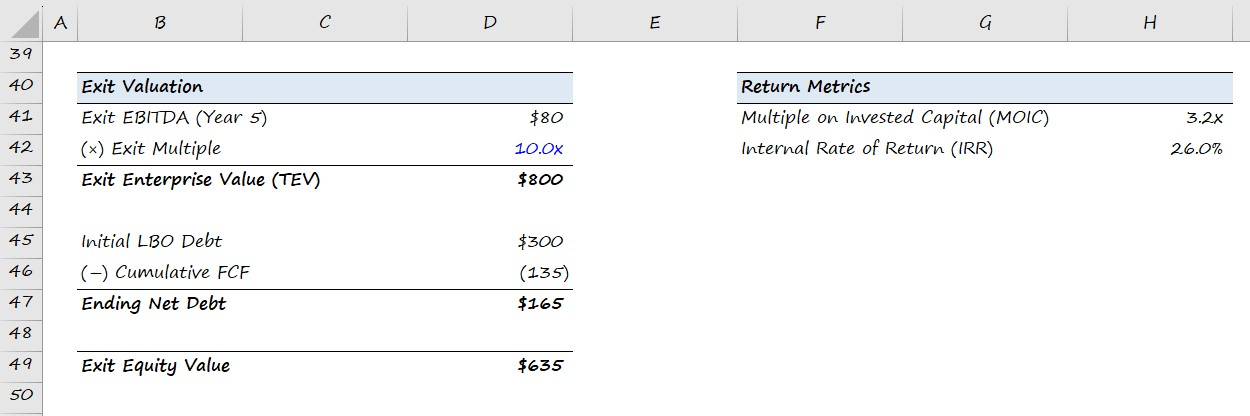Exit Enterprise Value (TEV) = Exit Year EBITDA × Exit Multiple
Cumulative FCFs = Σ Free Cash Flows (FCFs)
Final Year Net Debt = Initial Debt AmountCumulative FCFs
Exit Equity Value = Exit Enterprise ValueEnding Year Net Debt
Multiple on Invested Capital (MOIC) = Exit Equity Value ÷ Initial Sponsor Equity
Internal Rate of Return (IRR) = MOIC ^ (1 ÷ t) – 1
Master LBO Modeling Our Advanced LBO Modeling course will teach you how to build a comprehensive LBO model and give you the confidence to ace the finance interview.Inline FeedbacksArt
February 9, 2022 3:40 pm

Wouldn’t you acquire the company on an NTM EBITDA number?

Last edited 1 year ago by Arthur DussiasFebruary 10, 2022 2:39 pm

Hi, Art,

Projected EBITDA is the norm for Comps analysis, to be sure, but LBOs usually work off of LTM EBITDA, that way the purchase multiple and leverage multiple can reference the same number, and leverage multiples almost always use historical numbers, because they are actual numbers.Sarah
September 6, 2023 5:33 am

Hey, why did the D&A and Cap Ex not increase as a % of the increasing revenue? Why did the real figures stay constant at 5?Allison Clark
March 16, 2023 12:47 pm

Does this get you to levered or unlevered FCF?March 16, 2023 3:38 pm

Hi, Allison, Actually, it’s not strictly unlevered or levered FCF: not unlevered because we begin with net income rather than EBIT, and not levered because it is FCF before debt principal payments (levered FCF includes debt borrowing and payments); the way we should think about it is the FCF that… Read more »Joseph
June 4, 2022 10:53 am

I just completed this paper LBO – Thank you. In how long should this paper LBO need to have been completed?June 7, 2022 2:49 pm

Hi, Joseph,

The article says you should be able to arrive at the IRR in 5-10 minutes, so use that as a guide.

BB

X

The Wall Street Prep Quicklesson Series

7 Free Financial Modeling Lessons

Get instant access to video lessons taught by experienced investment bankers. Learn financial statement modeling, DCF, M&A, LBO, Comps and Excel shortcuts.